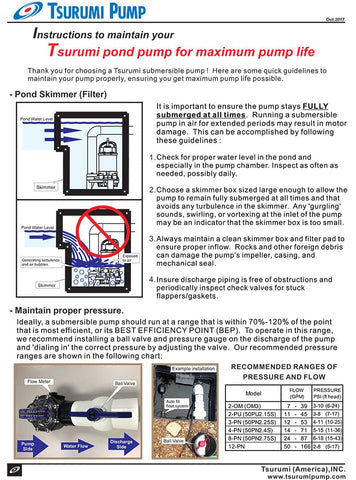# Tsurumi## TSURUMI'S GUIDE TO SIZING A WATERFALL PUMP

Determine the volume of a circular pond by measuring the diameter of the pond at its widest part and depth at its deepest part. Multiply the diameter by 2, then the depth, then by 5.9. For instance a circular pond with a diameter of 11 feet and a depth of 5 feet would have a volume of about 3,570 gallons (diameter x 2 x depth x 5.9 = volumne in gallons).

Calculate a square or rectangular pond's volume by measuring in feet and multiplying the length by the width by the depth measured at the deepest part by 7.5. For example , a pond that is 12 feet long, 11 feet wide and 5 feet deep would be about 4,950 gallons in volume (length x width x depth x 7.5 = volume in gallons).

Calculate the volume of an oval-shaped pond by measuring the length at its longest part, the width at the widest part and the depth at the deepest part. Multiply the length by the width by the depth by 6.7 to determine the volume. For instance, an ovular pond that is 11 feet long, 7 feet wide and 5 feet deep would have a volume of about 2,580 gallons (length x width x depth x 6.7 = volume in gallons)view all